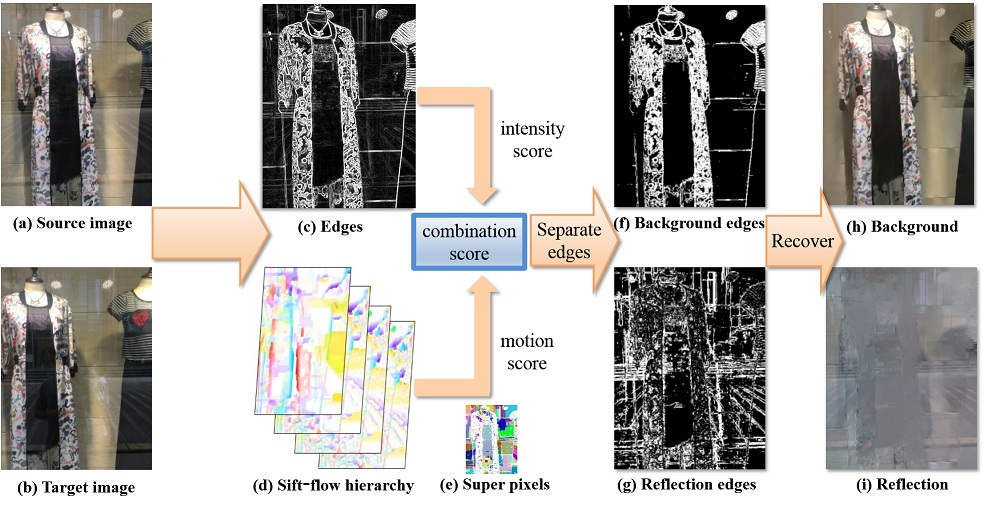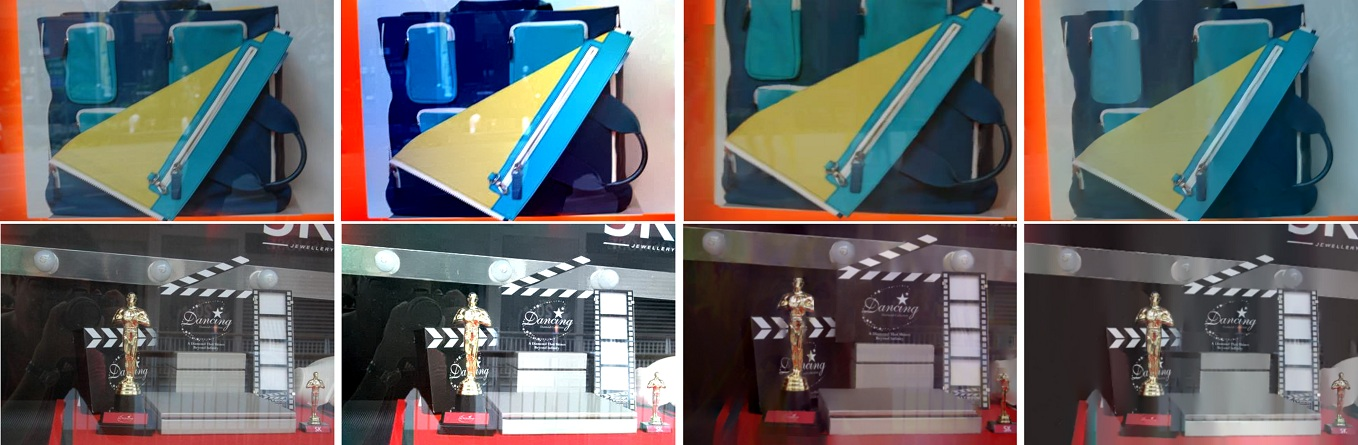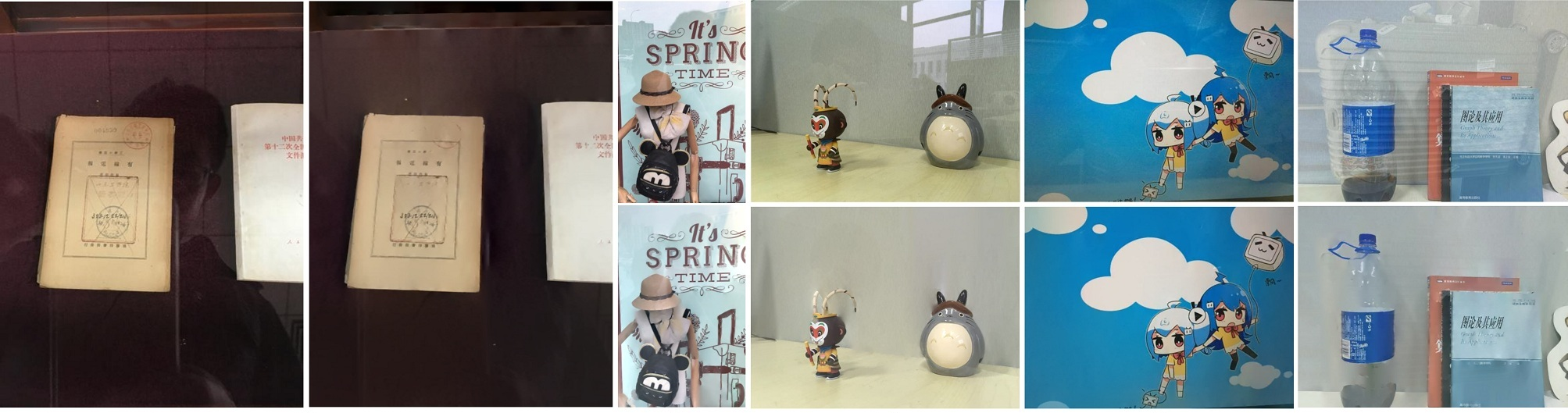﻿ Automatic Reflection Removal using Gradient Intensity and Motion Cues

# Automatic Reflection Removal using Gradient Intensity and Motion Cues

Chao Sun， Shuaicheng Liu， Taotao Yang Bing Zeng Zhengning Wang Guanghui Liu

University of Electronic Science and Technology of China

Abstract:

 We present a method to separate the background image and reflection from two photos that are taken in front of a transparent glass under slightly different viewpoints. In our method, the SIFT-flow between two images is first calculated and a motion hierarchy is constructed from the SIFT-flow at multiple levels of spatial smoothness. To distinguish background edges and reflection edges, we calculate a motion score for each edge pixel by its variance along the motion hierarchy. Alternatively, we make use of the so-called superpixels to group edge pixels into edge segments and calculate the motion scores by averaging over each segment. In the meantime, we also calculate an intensity score for each edge pixel by its gradient magnitude. We combine both motion and intensity scores to get a combination score. A binary labelling (for separation) can be obtained by thresholding the combination scores. The background image is finally reconstructed from the separated gradients. Compared to the existing approaches that require a sequence of images or a video clip for the separation, we only need two images, which largely improves its feasibility. Various challenging examples are tested to validate the effectiveness of our method
 Paper [PDF (7.6MB)]
 PipeLinePipeline of our system. (a) and (b) are the source and target input images. (c) An intensity score is derived from gradient edges. (d) SIFT-flow hierarchy is computed with di erent levels of smoothness, which produces motion scores for edge segments that are grouped by image superpixels (shown in (e)). We combine two scores to separate the edges into (f) reflectance edges and (g) background edges. (h) The final background image is recovered through the background edges. Optionally, a reflectance image (i) can also be reconstructed.